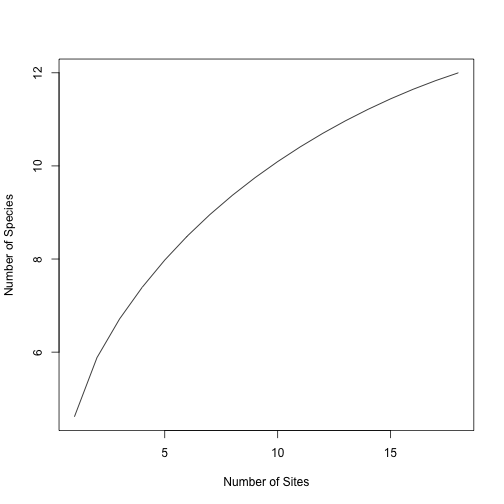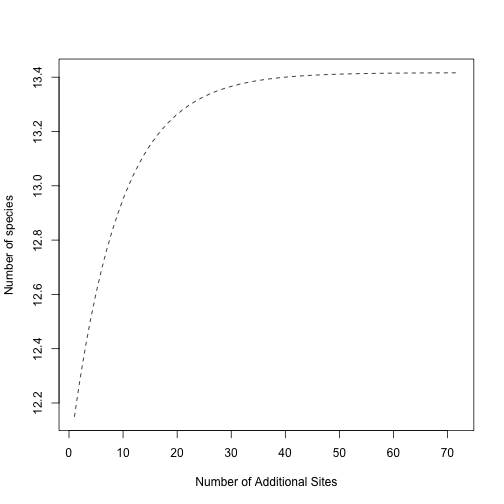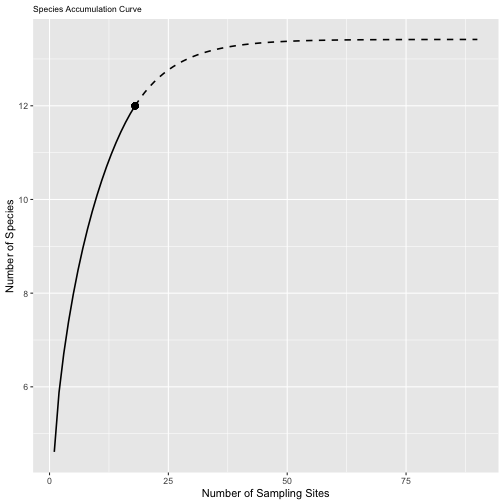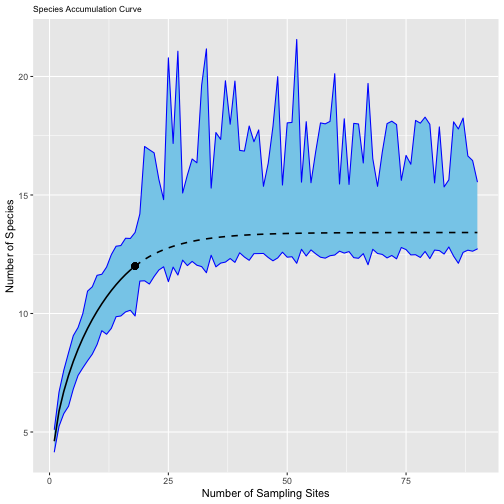# Background

This is a guest post written by Joseph Mack

This post, written by Joseph Mack, explores the working of the unified
interpolation/extrapolation tools described in Colwell et al. 2012 Journal of
Plant Ecology
“Models and estimators linking individual-based and sample-based
rarefaction, extrapolation and comparison of assemblages” doi:
10.1093/jpe/rtr044. Joseph shared this tutorial with the Fall 2021 Biodiversity
Measurement seminar.

# Joseph’s tutorial

First, we must import a sample presence-absence dataset.

``````spp_incidence <- read.csv("data/ann_incidence.csv", row.names = 1)
``````

The equations used in this analysis are derived from a Bernoulli Product model.
Under this model, species \(i\) has probability theta of occurring. What is the
probability that species \(i\) is not present in a given site?

Do we know this probability for each species in our dataset?

To proceed, we must determine how many species (`Sobs`) and sites (`sites`) are
in the data set (`Sobs`)?

``````sites <- length(spp_incidence)
Sobs <- nrow(spp_incidence)
sites
``````
```##  18
```
``````Sobs
``````
```##  12
```

The equation below can be found on pg. 6 of Colwell et al. 2012. It calculates
the incidence-based frequency of a given species.

``````#What is the incidence-based frequency (Yi) of Sp1?
Ysp1 = sum(as.numeric(spp_incidence["sp1",]))
Ysp1
``````
```##  NA
```
``````#What about the other species?
spp_names = rownames(spp_incidence)
Yi <- c(1:12)
for (i in 1:12) {
Yi[i] <- sum(spp_incidence[i,])
}
``````

The code below creates a table of incidence frequencies (the row sums of our
data set), which will be used to calculate `Qk`.

``````incidence_freqs <- data.frame(cbind(Yi, spp_names))
``````

Next, we must determine how many ‘unique’ species are in the dataset. In other
words, how many species occur in only one site? This requires us to calculate
`Qk`, the incidence frequency count and an essential statistic for sample-based
extrapolation.

``````Q <- function(k) {
out = 0
for (i in 1:length(incidence_freqs\$Yi)) {
if (incidence_freqs\$Yi[i] == k) {
out = out + 1
}
}
return(out)
}

#How many species are found in only 1 site? 4 sites? 9 of the sites? All the sites?
Q(1)
``````
```##  3
```
``````Q(4)
``````
```##  1
```
``````Q(9)
``````
```##  0
```
``````Q(18)
``````
```##  1
```

Below is a function to interpolate/rarefy the sample dataset (equation 17 in
Colewll et al.). It computes the number of species we would recover if we
sampled from `x` fewer sites (`x < sites`).

``````Ssample_rare <- function(x) {
for (i in 1:Sobs) {
out <- (choose((sites - as.numeric(incidence_freqs\$Yi[i])), x))/
choose(sites, x)
}
return(Sobs - sum(out))
}

#How many species would we recover if we sampled from only 1 site? 5 sites? 9 sites?
Ssample_rare(1)
``````
```##  11.05556
```
``````Ssample_rare(5)
``````
```##  11.27778
```
``````Ssample_rare(9)
``````
```##  11.5
```

The values for `Ssample_rare` don’t look right. This rarefaction function is
broken! Can you figure out why? Hint: what is `[i]` doing in the code below?

The rarefaction code below works! Why?

``````Ssample_rare <- function(x) {
out = c()
for (i in 1:Sobs) {
out[i] <- (choose((sites - as.numeric(incidence_freqs\$Yi[i])), x))/choose(sites, x)
}
return(Sobs - sum(out))
}

#NOW, how many species would we recover if we sampled from only 1 site? 5 sites? 9 sites?
Ssample_rare(1)
``````
```##  4.611111
```
``````Ssample_rare(5)
``````
```##  7.977708
```
``````Ssample_rare(9)
``````
```##  9.748416
```
``````#These values make more sense!
``````

Now that we have a means to interpolate our data, we can make a rarefaction plot.

``````diversity_r = sapply(1:18, Ssample_rare) # This outputs a vector of Ssample_rare
# outputs for 1 to 18 sampling sites.
plot(1:18
, diversity_r
, type = "l"
, ylab = "Number of Species"
, xlab = "Number of Sites")
``````Next, we can work on extrapolating the dataset `(Ssample(sites + s))` using
equations 18 and 21 from Colwell et al. 2012. This addresses how many species we
would find if we sampled `s` additional sites.

To do this, we first need to estimate `Q(0)`: the number of species absent from
our samples.

``````#Because Q(2) > 0 for our data set, we can use the following equation based on the Chao2 estimator:
Q0 = ((sites-1)/sites)*((Q(1)^2)/(2*Q(2)))
Q0
``````
```##  1.416667
```

With a value for `Q0`, we can implement the authors’ equation for extrapolation
as a function of `s` additional samples:

``````Ssample_extr <- function(s) {
out <- Sobs + (Q0*(1 - (1 - (Q(1)/(Q(1) + (sites*Q0))))^s))
return(out)
}

#How many species would we recover if we sampled 2 extra sites? 50 extra sites?
Ssample_extr(2)
``````
```##  12.28255
```
``````Ssample_extr(50)
``````
```##  13.41122
```
``````#Make an extrapolation plot for 72 additional sites.
diversity_e = sapply(1:72, Ssample_extr) #This outputs a vector of Ssample_extr
# outputs for 1 to 50 additional sampling sites.
plot(1:72
, diversity_e
, type = "l"
, lty = 2
, ylab = "Number of species"
, xlab = "Number of Additional Sites")
``````Now, we can combine the rarefaction and extrapolation plots into a single plot:

``````library(ggplot2)
combined_diversity = c(diversity_r, diversity_e) #Combining the vectors of
# rarefied and extrapolated species counts, for 1 - 90 sites.
combined_diversity
``````
```##    4.611111  5.882353  6.713235  7.391830  7.977708  8.494344  8.955505  9.371041  9.748416 10.093263 10.409754 10.700927
##  10.968954 11.215359 11.441176 11.647059 11.833333 12.000000 12.149123 12.282548 12.401929 12.508744 12.604315 12.689825
##  12.766335 12.834791 12.896041 12.950844 12.999878 13.043750 13.083005 13.118127 13.149552 13.177669 13.202827 13.225337
##  13.245477 13.263497 13.279620 13.294046 13.306953 13.318502 13.328835 13.338081 13.346353 13.353754 13.360377 13.366302
##  13.371603 13.376347 13.380591 13.384389 13.387786 13.390826 13.393546 13.395980 13.398158 13.400106 13.401849 13.403409
##  13.404804 13.406053 13.407170 13.408170 13.409064 13.409865 13.410581 13.411221 13.411794 13.412307 13.412766 13.413177
##  13.413544 13.413873 13.414167 13.414430 13.414665 13.414876 13.415065 13.415233 13.415384 13.415519 13.415640 13.415748
##  13.415845 13.415931 13.416009 13.416078 13.416140 13.416195
```
``````sampling_sites = 1:90 #vector for 18 rarefied sites + 72 additional extrapolated
# sites
sampling_sites
``````
```##    1  2  3  4  5  6  7  8  9 10 11 12 13 14 15 16 17 18 19 20 21 22 23 24 25 26 27 28 29 30 31 32 33 34 35 36 37 38 39 40 41 42
##  43 44 45 46 47 48 49 50 51 52 53 54 55 56 57 58 59 60 61 62 63 64 65 66 67 68 69 70 71 72 73 74 75 76 77 78 79 80 81 82 83 84
##  85 86 87 88 89 90
```
``````diversity_est <-
data.frame(cbind(combined_diversity, sampling_sites))
sac_plot = ggplot(
data = subset(diversity_est, sampling_sites <= 18),
aes(x = sampling_sites, y = combined_diversity)
) +
geom_line(size = 0.75) +
geom_line(
data = subset(diversity_est, sampling_sites >= 18),
aes(x = sampling_sites, y = combined_diversity),
lty = 2,
size = 0.75
) +
geom_point(aes(x = 18, y = 12), size = 3, pch = 16) +
xlab("Number of Sampling Sites") +
ylab("Number of Species") +
ggtitle("Species Accumulation Curve") +
theme(plot.title = element_text(size = 8.5))
``````
``````sac_plot
``````What does the point represent in this plot? The dotted line? The solid line? Is
our data set a good approximation for the total number of species in the region
sampled?

It is possible to obtain a 95% confidence interval about the species
accumulation curve. However, the variance estimators provided by Colwell et al.
2012 are difficult to understand. As an alternative, we can employ
non-parametric bootstrapping to get 95% confidence intervals about the curve.
The code below is not explicitly described in Colwell et al. 2012, but it
employs the same estimators we used above.

Below is a function for getting bootstrap replicates of our rarefaction and
extrapolation estimates.

``````Ssample_boot <- function(x, s) {
# First, we need to build matrices and vectors to hold our boot-strapped data replicates.
boot_data <- matrix(nrow = 12, ncol = 18)
Yi_boot <- c(1:12)
for (i in 1:12) {
# The line below creates a replicate dataset from our original spp_incidence dataset,
# with replacement.
boot_data[i, ] <- rbind(as.numeric(sample(spp_incidence[i, ]
, size = 18
, replace = TRUE)))
#The for loop below calculates the incidence frequencies from the replicated dataset.
for (j in 1:12) {
Yi_boot[j] <- sum(boot_data[j,])
}
}
# Below is the equation for rarefaction that we implemented earlier, where x is
# a sample smaller than sites.
out_rare = c()
for (i in 1:Sobs) {
out_rare[i] <- choose(sites - Yi_boot[i], x)/choose(sites, x)
}

#This calculates a value of Q2 for the replicate data set.
Q2 = sum(Yi_boot == 2)

#This calculates a value of Q1 for replicate data set.
Q1 = sum(Yi_boot == 1)

# We are using a different equation for Q0 here (Equation 22),
# to avoid dividing by 0 when Q2 is 0 in some of our replicates.
Q0_b = ((sites - 1)/sites) * (Q1 * ((Q1 - 1))/(2 * (Q2 + 1)))

# Below is just equation 18, with some special conditions to account for
# scenarios where the function divides by 0.
out_extr <- Q0_b * (1 - (exp((-s * Q1))/(Q1 + sites * (Q0_b))))
if (is.nan(out_extr) == TRUE) {
return(c(Sobs - sum(out_rare), Sobs))
} else {
return(c(Sobs - sum(out_rare), Sobs + out_extr))
}
}
``````

The code below tests that the function is working. The first variable (`x`)
specifies the sample size to be interpolated/rarefied, while the second variable
(`s`) specifies the additional sites to be extrapolated. The output should be
different each time you run it.

``````Ssample_boot(1,2)
``````
```##   4.388889 12.000000
```

The code below gets bootstrap replicates using `replicate` and `mapply`. How
many bootstrap replicates are we running?

``````var1 <- 1:18
var2 <- 1:90
boot_data <- replicate(100, mapply(Ssample_boot, var1, var2))
``````

Now that we have our bootstrap replicates, we can set up an empty matrix to
store our bootstrap replicate data. Each column is a site (1 – 18 interpolated,
19 – 90 extrapolated), and each row is a bootstrapped replicate.

``````combined_boot_data <- matrix(ncol = 90, nrow = 100)

#The code below populates the matrix.
for (i in 1:100) {
combined_boot_data[i, 1:18] <- boot_data[, , i][1, 1:18]
}
for (i in 1:100) {
combined_boot_data[i, 19:90] <- boot_data[, , i][2, 19:90]
}
combined_boot_data <- data.frame(combined_boot_data)
``````

Now that we have bootstrapped replicates for rarefaction and extrapolation, we
can estimate mean, 2.5% , and 97.5% quantiles for each site (1 – 18 rarefied, 19

• 90 extrapolated). These values can then be used to estimate a 95% confidence
interval centered about our sample data.
``````#This creates an empty vector to store the means of our bootstrapped data.
boot_means <- c()

#This estimates the average across 100 bootstrap replicates for each site (1 - 90).
for (i in 1:90) {
boot_means[i] = (sum(combined_boot_data[,i]))/100
}

#This creates an empty data frame to store the 2.5% and 97.5% quantiles of our bootstrapped data.
boot_quantiles <- matrix(nrow = 2, ncol = 90)
boot_quantiles <- data.frame(boot_quantiles)

# This computes the 2.5% and 97.5% quantiles of our data set,
# populating the data frame we created above.
for (i in 1:90) {
boot_quantiles[,i] <- unname(quantile(combined_boot_data[,i], probs = c(0.025, 0.975)))
}
CI_data <- rbind(combined_boot_data, boot_means, boot_quantiles)
``````

With the average, 2.5%, and 97.5% quantiles, we can compute the lower and upper
confidence bounds for each rarefied and extrapolated site.

For each site, the lower confidence bound = sample data – (bootstrap average –
2.5% bootstrap quantile).

``````LCB <- c()
for (i in 1:90) {
LCB[i] <- combined_diversity[i] - (CI_data[101,i] - CI_data[102,i])
}
``````

For each site, the upper confidence bound = sample data + (97.5% bootstrap
quantile – bootstrap average).

``````UCB <- c()
for (i in 1:90) {
UCB[i] <- combined_diversity[i] + (CI_data[103,i] - CI_data[101,i])
}
confidence_intervals <- data.frame(cbind(LCB,UCB))
``````

With this, we can plot 95% confidence intervals about the species accumulation
curve using the LCB and UCB values calculated above. The block of code below is
mostly identical to the plotting section above. What did we change?

``````sampling_sites = 1:90 #18 sites + 72 additional sites
diversity_est <-
data.frame(cbind(combined_diversity, sampling_sites))
sac_plot = ggplot(
data = subset(diversity_est, sampling_sites <= 18),
aes(x = sampling_sites, y = combined_diversity)
) +
geom_ribbon(
confidence_intervals,
mapping = aes(ymin = LCB, ymax = UCB),
fill = "skyblue",
col = "blue"
) + #Use geom_ribbon to plot our upper and lower confidence intervals.
geom_line(size = 0.75) +
geom_line(
data = subset(diversity_est, sampling_sites >= 18),
aes(x = sampling_sites, y = combined_diversity),
lty = 2,
size = 0.75
) +
geom_point(aes(x = 18, y = 12), size = 3, pch = 16) +
xlab("Number of Sampling Sites") +
ylab("Number of Species") +
ggtitle("Species Accumulation Curve") +
theme(plot.title = element_text(size = 8.5))
sac_plot
``````